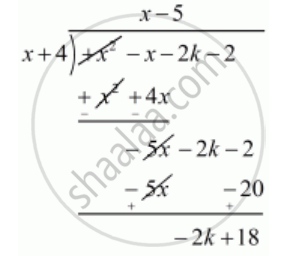Advertisement Remove all ads

# For What Value of K, −4 is a Zero of the Polynomial X2 − X − (2k + 2)? - Mathematics

Sum

For what value of k, −4 is a zero of the polynomial x2 − x − (2k + 2)?

Advertisement Remove all ads

#### Solution

We know that if  x = a is zero polynomial then  x - 2 is a factor of f(x)

Since  -4  is zero of  f(x)

Therefore  x+ 4  is a factor of  f(x)

Now, we divide f(x)= x^ -x -(2 k+ 2 ) by   g(x)= x+ 2 to find the value ofNow, Remainder  = 0

 -2k + 18 =0

 - 2k = -18

 k = (-18)/(-2)

k = (cancel(-)18)/(cancel(-)2)

k = 9

Hence, the value of k is  9

Is there an error in this question or solution?
Advertisement Remove all ads

#### APPEARS IN

RD Sharma Class 10 Maths
Chapter 2 Polynomials
Q 29 | Page 60
Advertisement Remove all ads

#### Video TutorialsVIEW ALL 

Advertisement Remove all ads
Share
Notifications

View all notifications

Forgot password?